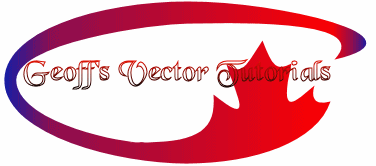Using Vectors

First of all you need to know the difference between vector and raster.
Here is as simple an explanation as I can give.
A raster graphic could be compared to a painting or drawing on paper.
A vector graphic is a mathematical formula from which a drawing is made.
Obviously a raster graphic can be manipulated a little but edges become less defined when any change is made. A vector graphic is changed mathematically so a new drawing is made each time a change is made.
This keeps edges crisp like the original.
I will use one of the preset shapes and fill it with a gradient for an example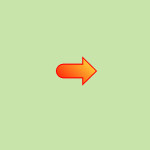We must presume that this is a bit more complex to see the advantages but without being made as a vector we cannot change the color, fill or width of the outer line.
 All we can do is resize and this is what we get.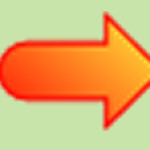If we resize the vector it comes out like this.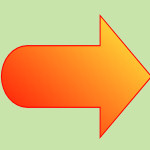We have complete control of the vector graphic and can change all aspects to anything we like.
There is much more to vectors if you like to draw your own shapes. For instance an egg shape where one end is more pointed than the other can be acheived in seconds but is extremely difficult to draw without using the vector tools.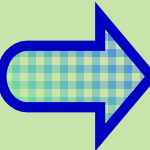Home